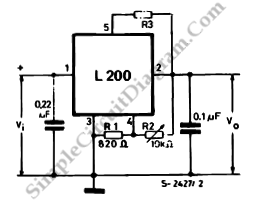# Programmable Current and Voltage Regulator for Battery Charger

This is a programmable current and voltage regulator circuit. It consist of a voltage regulator with external current limitation. The variable resistor R2 is forced by a constant current because of the fixed voltage of 2.77 V at the terminals of R1. The voltage at pin 2 and the output voltage are varied, if R2 is varied. Here is the circuit:The value of R1 should be kept low, to maintain a sufficient current for good regulation. The output current is Vref/R1,  when there is no load. When there is the load, the current value (10 µA) at the input of the error amplifier (pin 4) limits the maximum value for R1.

The output voltage of this circuit is :
vo=Vref*(1+(R2/R1)) with Vref=2.77 V, The maximum output current is:
Iomax=V5-2/R3 with V5-2=0.45 V

This regulator can be used to charge almost any battery type: by setting the output voltage at the battery’s voltage (fully charged condition), and setting the current limiter at 0.5 to 0.75 battery capacity/hour. [Source: STMicroelectronics Application Note]